# RS Aggarwal Solutions for Class 12 Maths Chapter 10: Differentiation Exercise 10B

Derivatives of exponential and logarithmic functions are the important topics which are explained under this exercise. Students who find it difficult in solving the exercise wise problems can download the PDF of solutions and use it as a reference guide. The problems are solved step by step with explanations, according to the understanding capacity of students. For a better hold on these concepts, students can access RS Aggarwal Solutions Class 12 Maths Chapter 10 Differentiation Exercise 10B PDF, from the links given below.

## RS Aggarwal Solutions for Class 12 Maths Chapter 10: Differentiation Exercise 10B Download PDF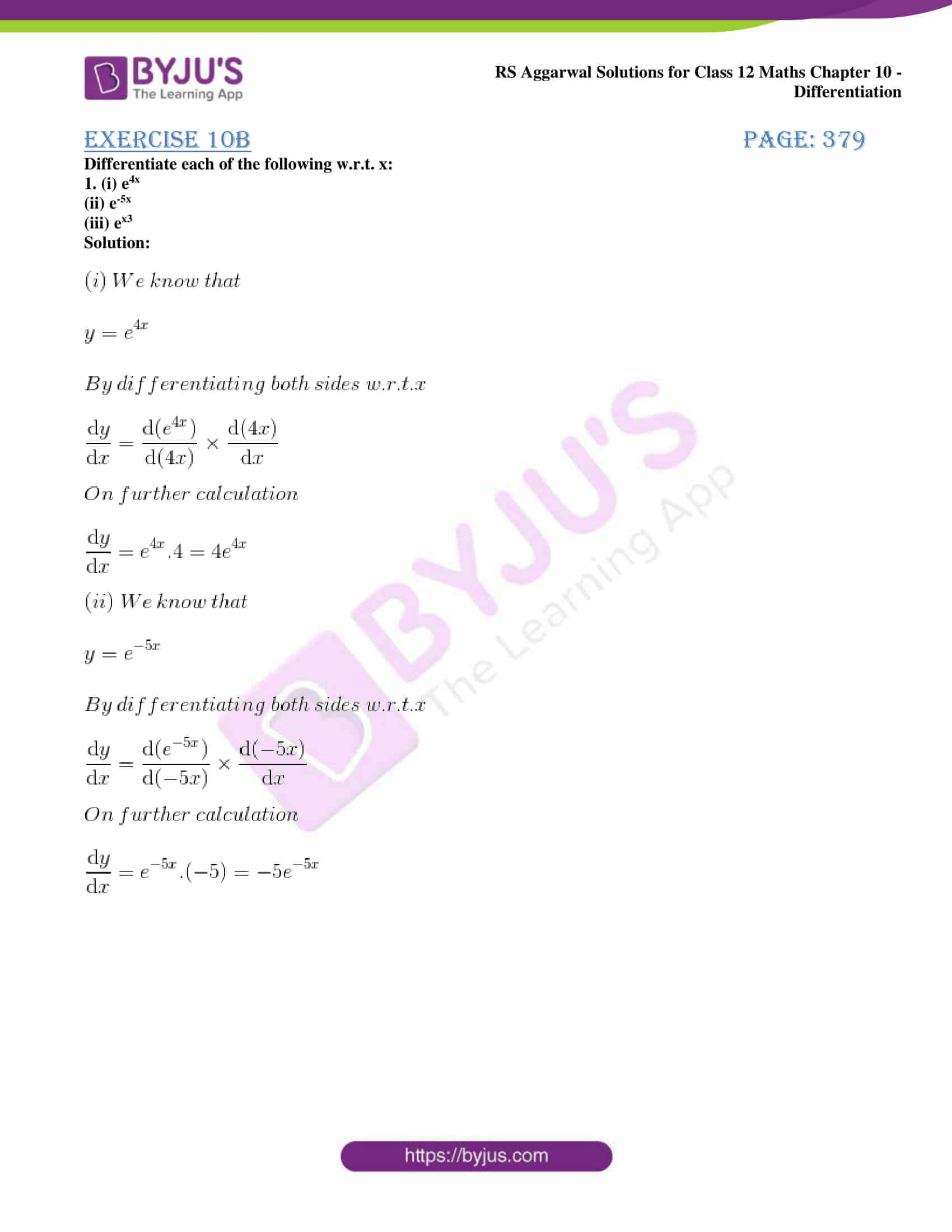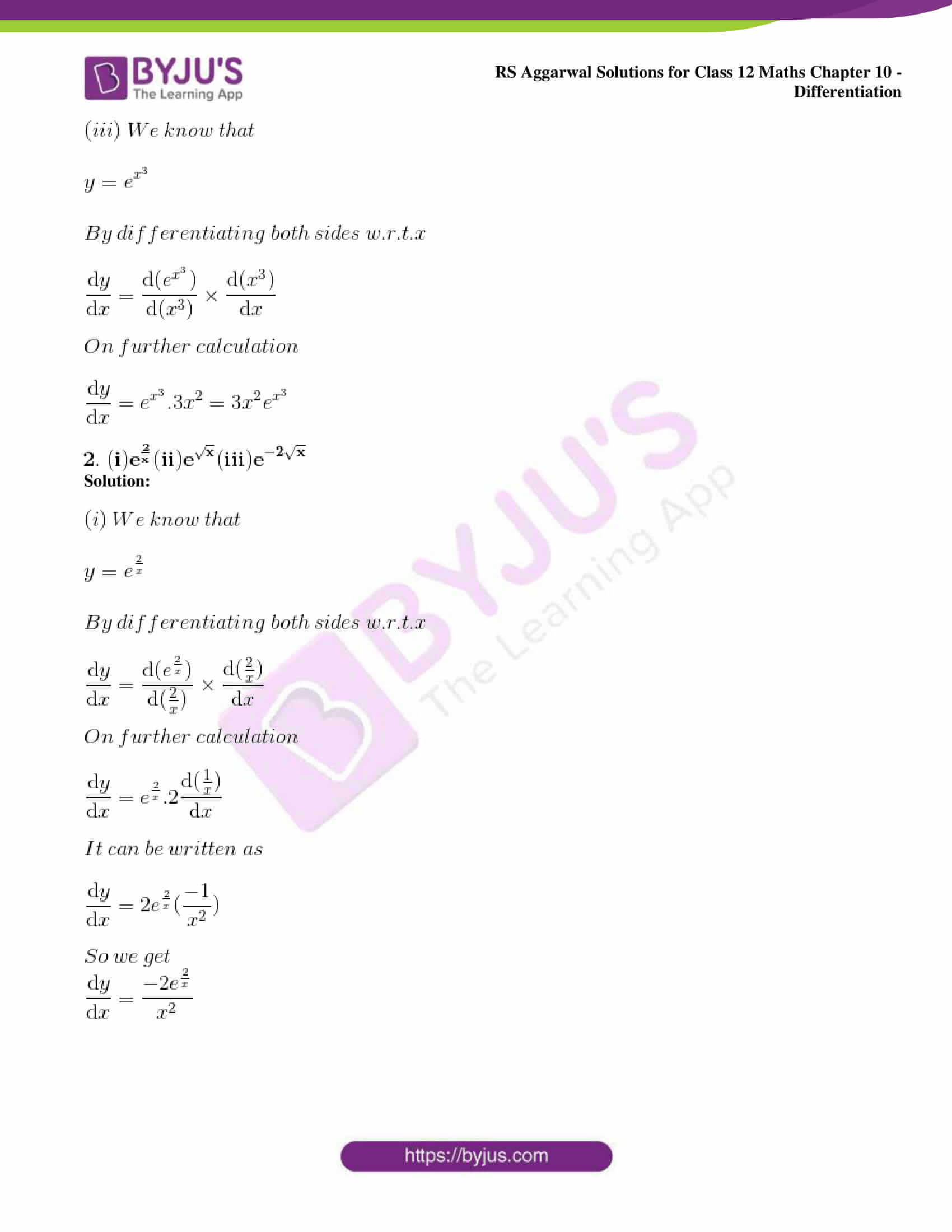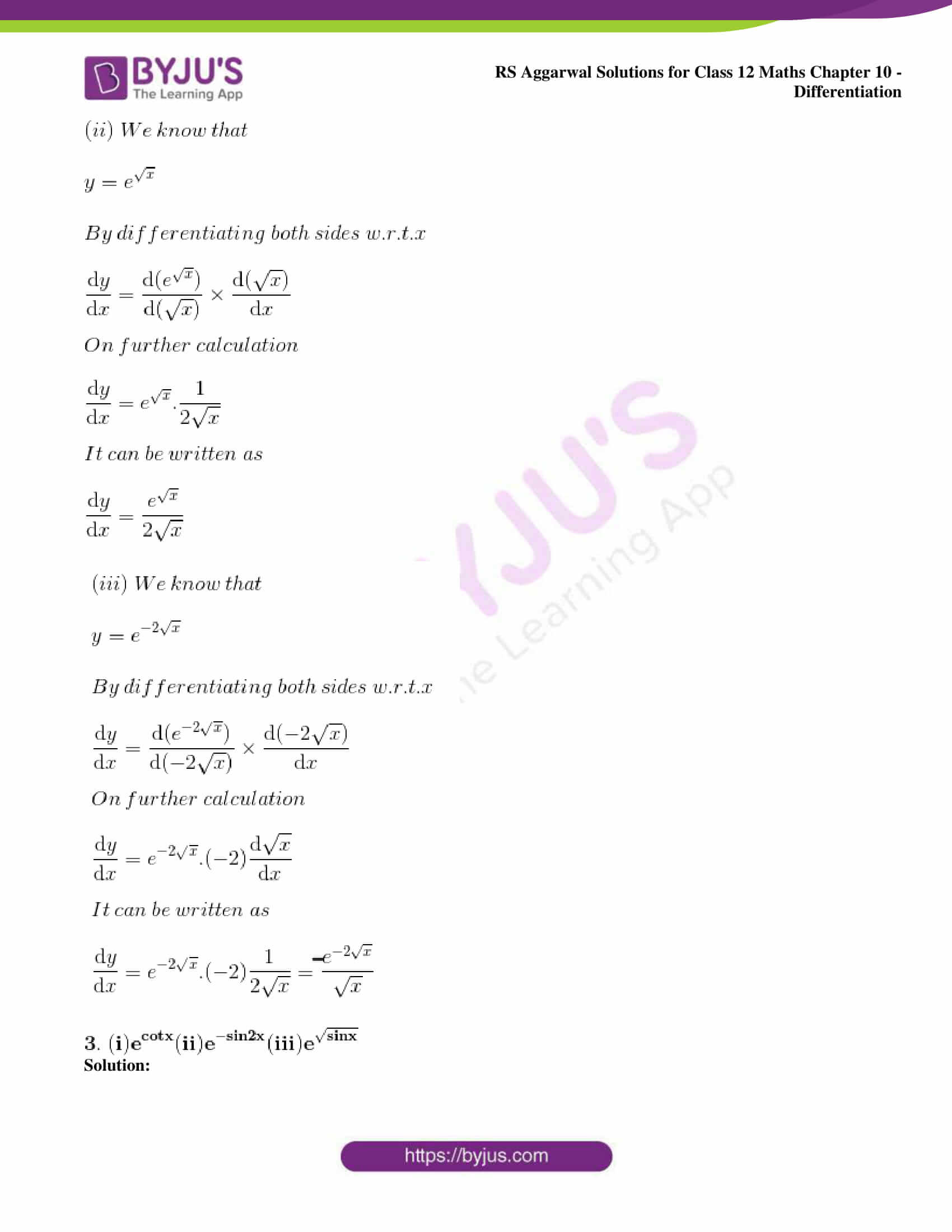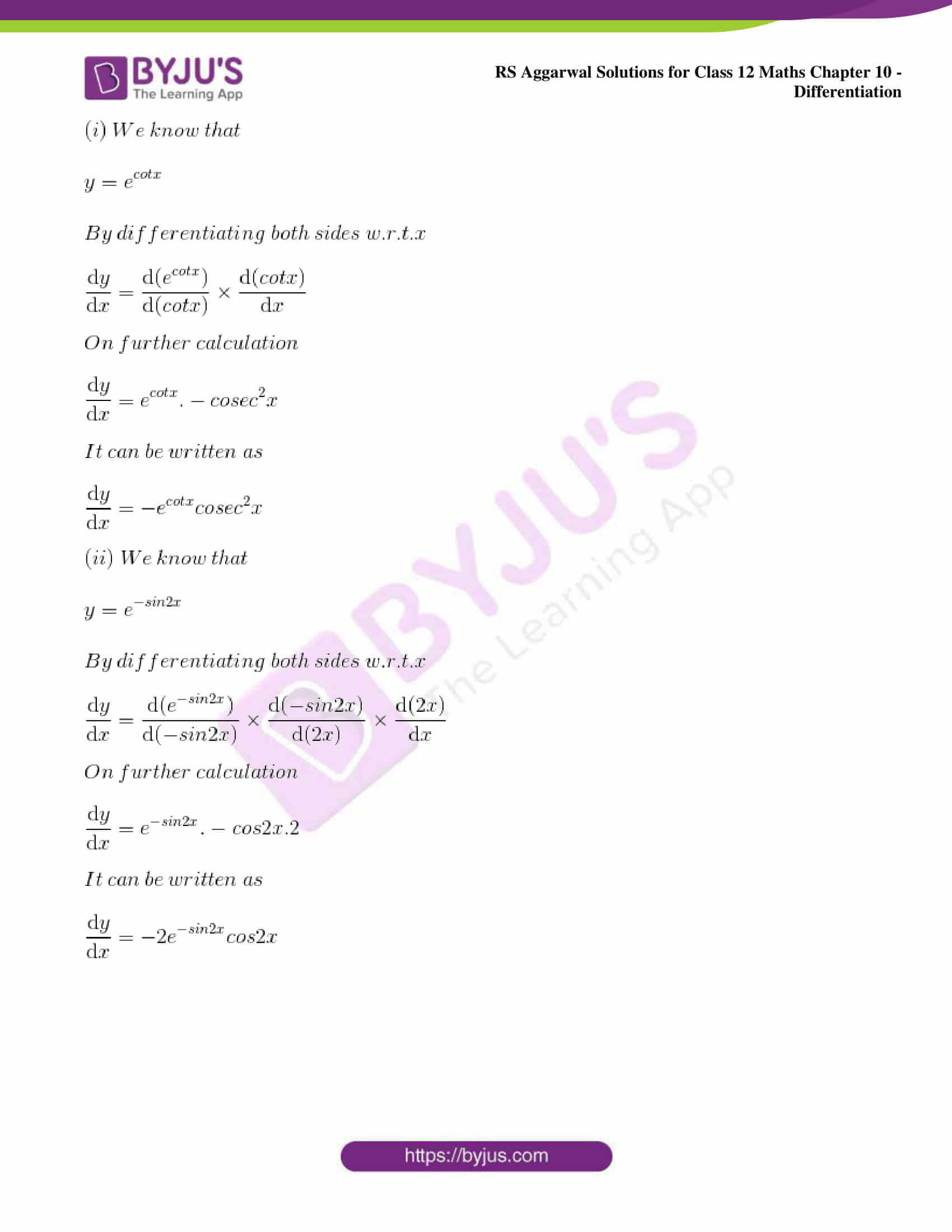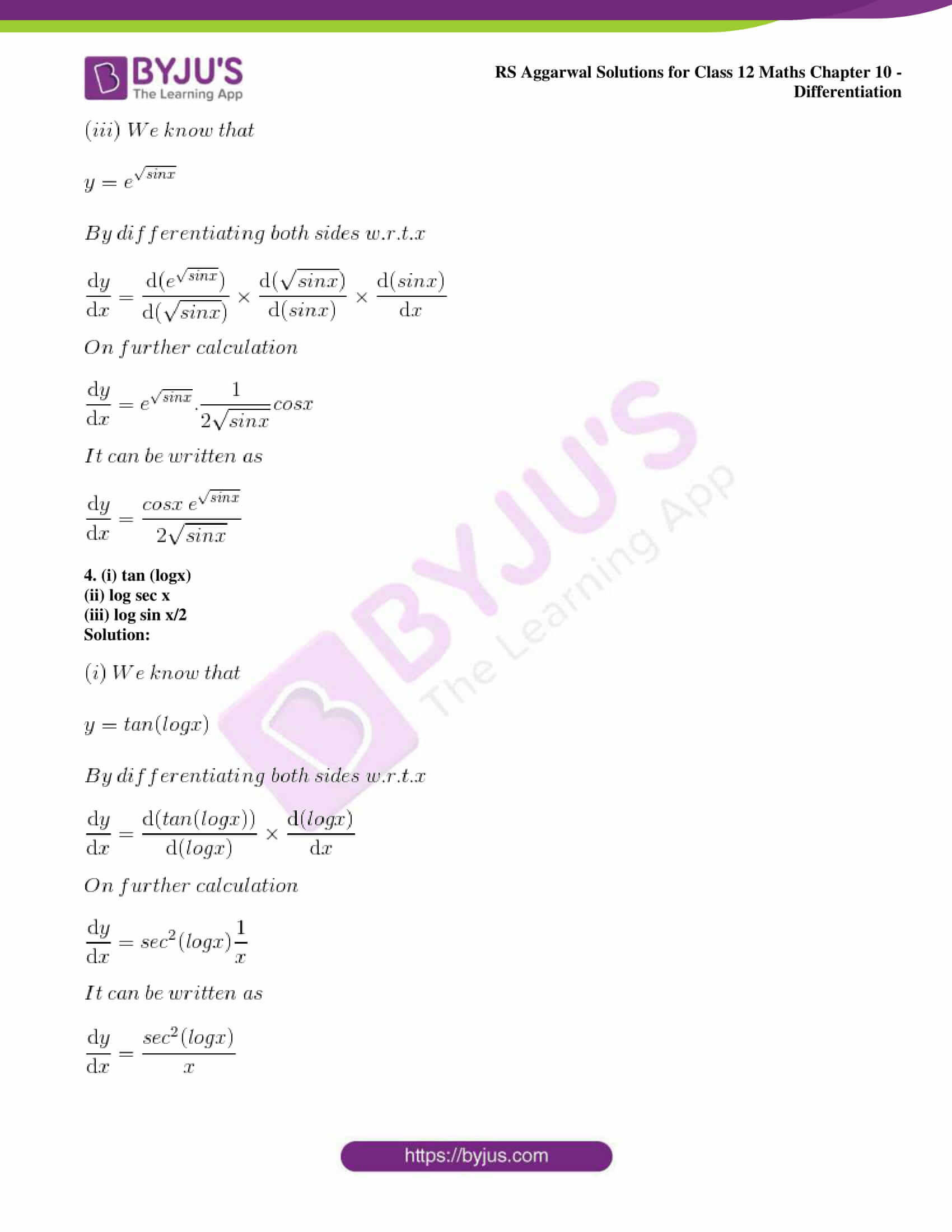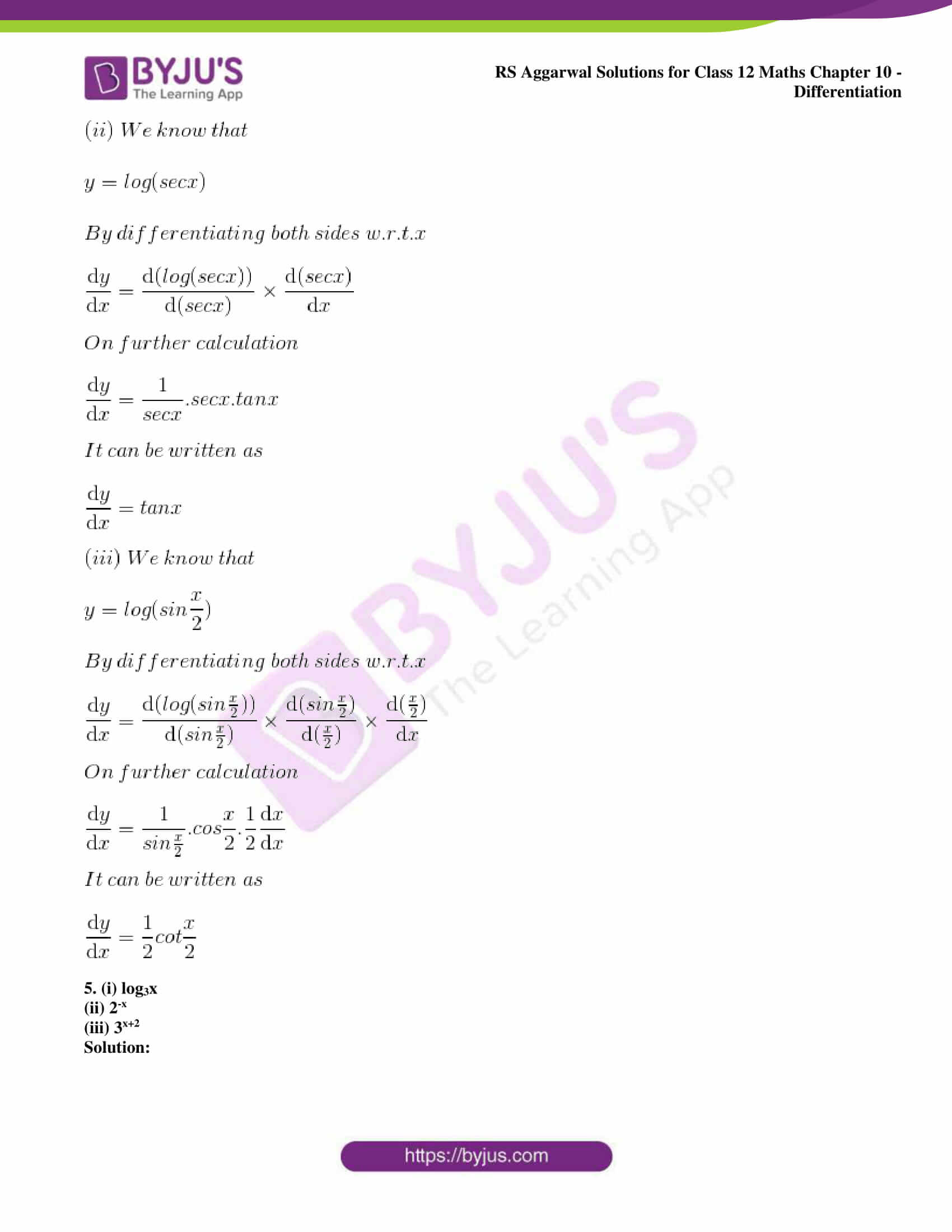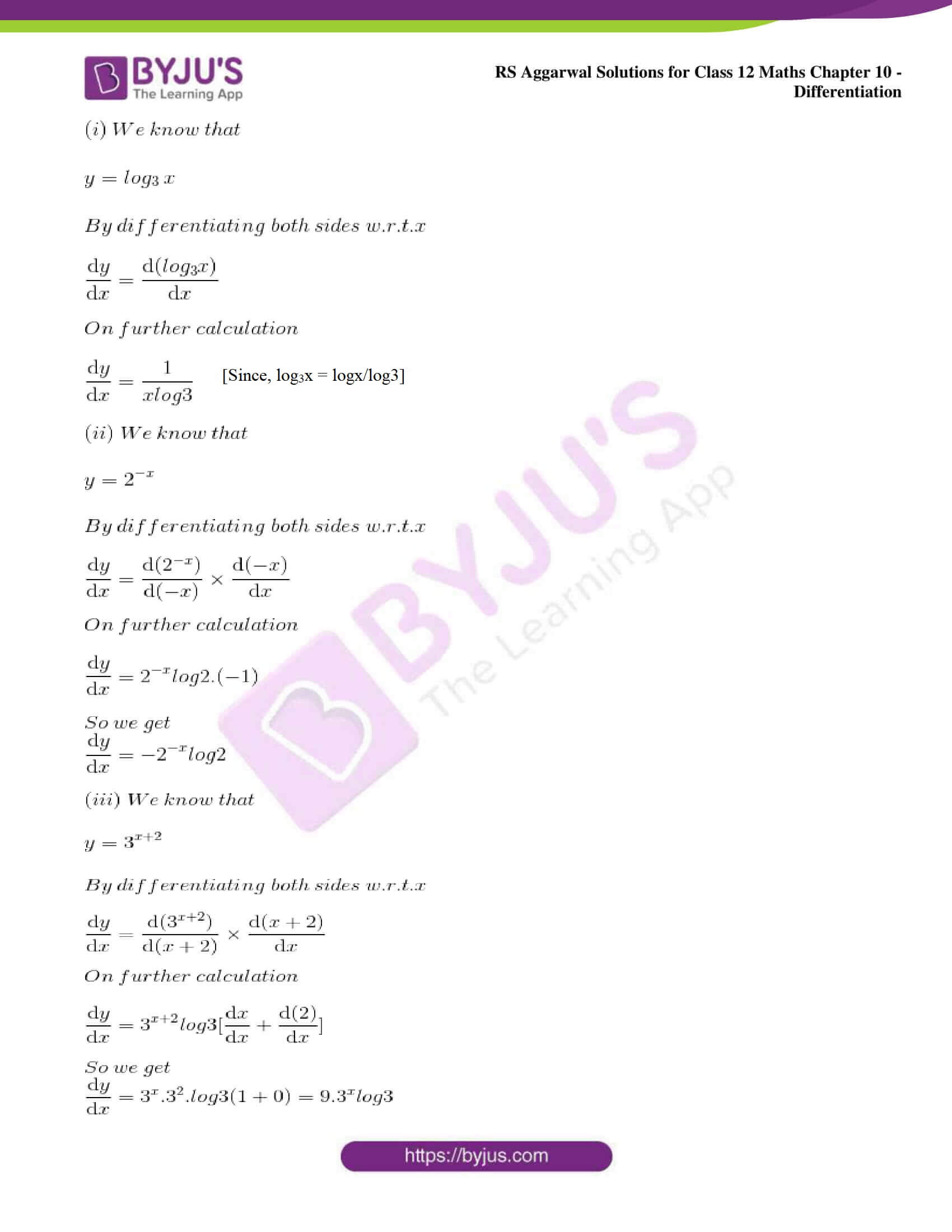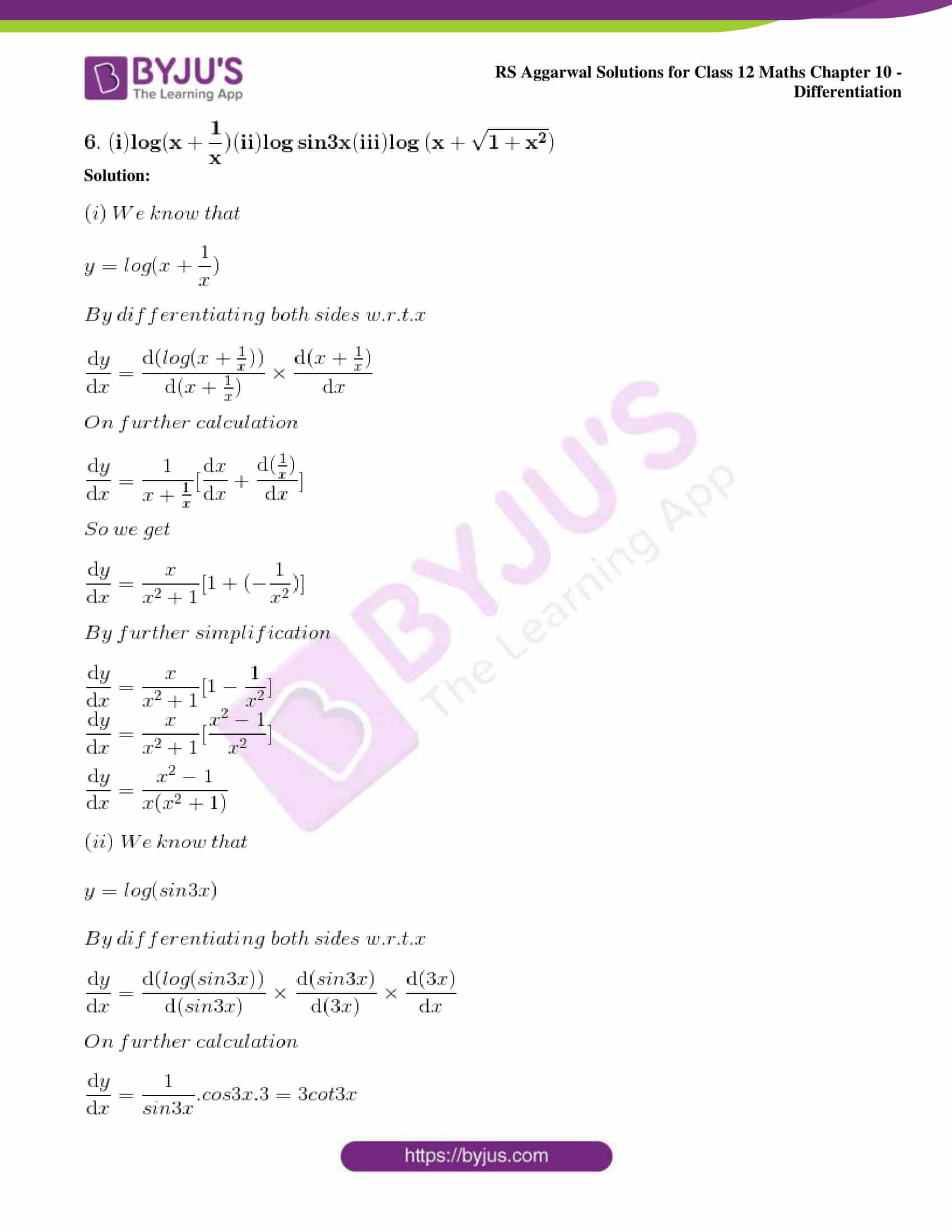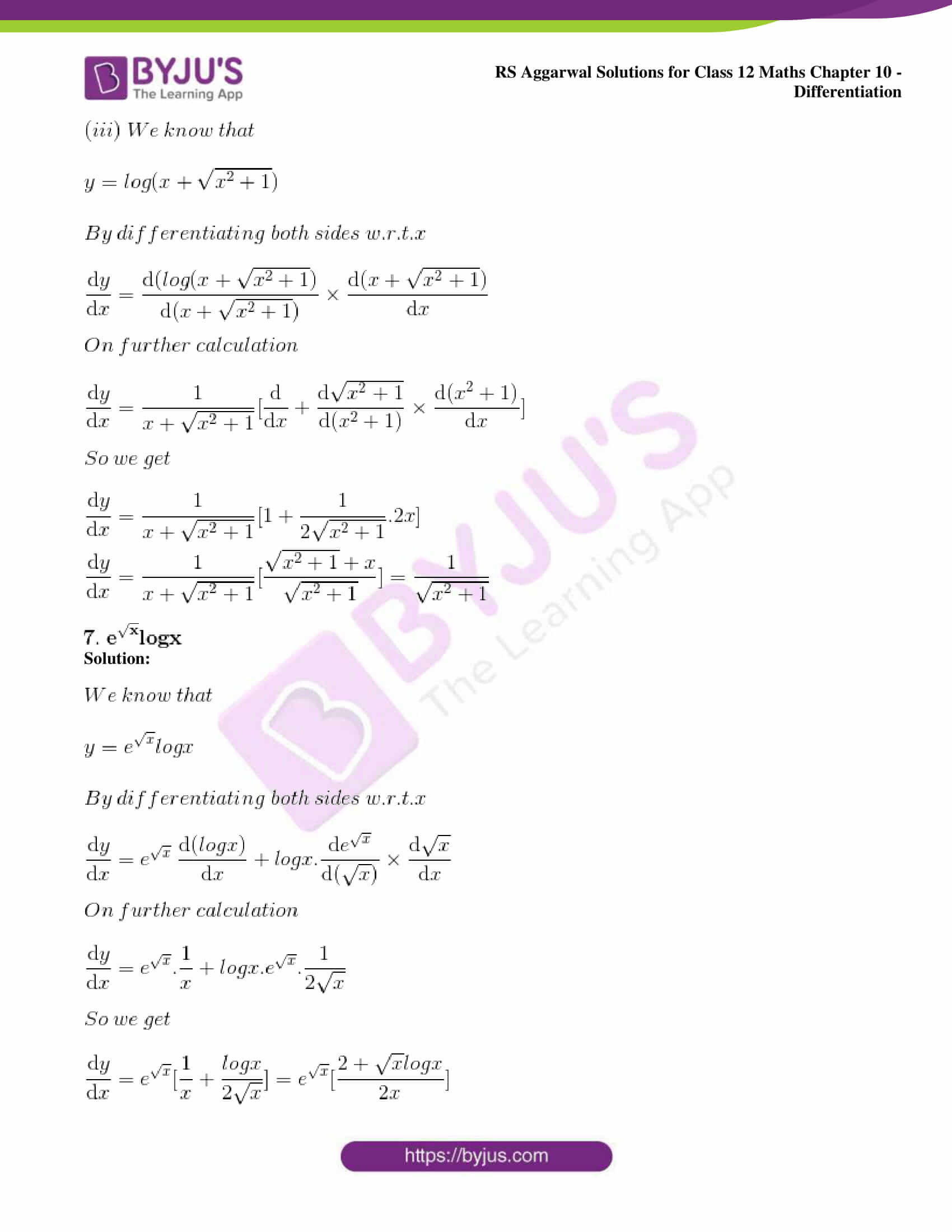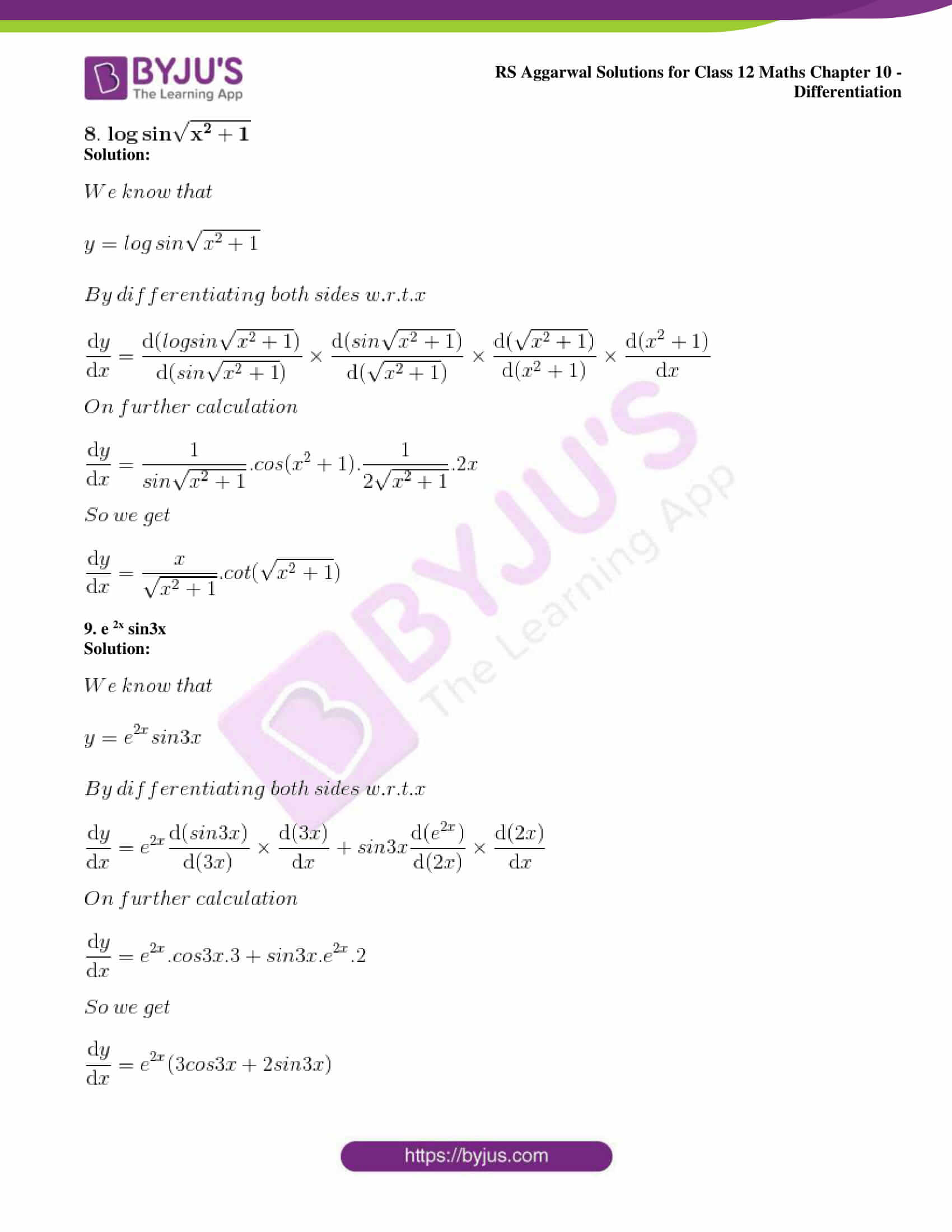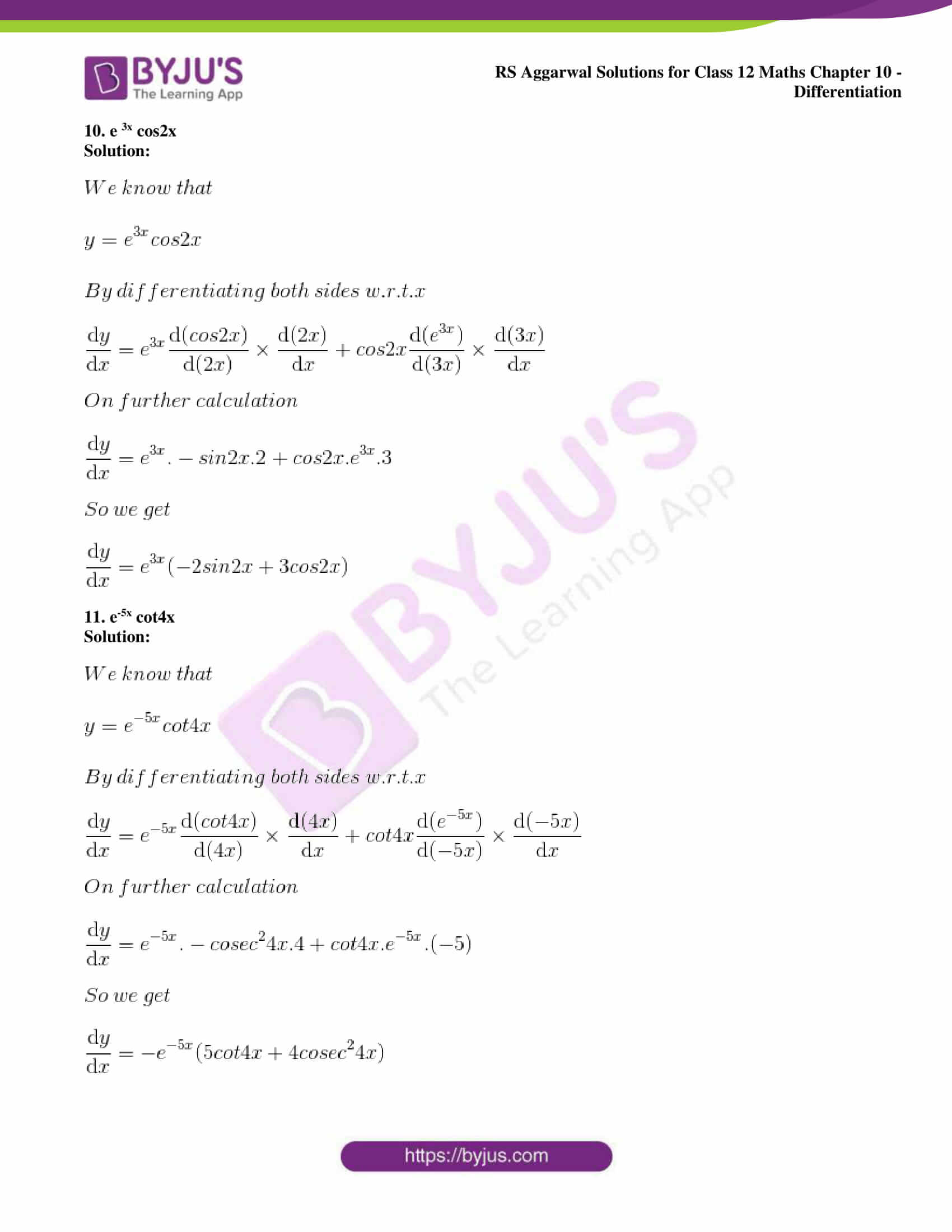### Access other exercise solutions of Class 12 Maths Chapter 10: Differentiation

Exercise 10A Solutions

Exercise 10C Solutions

Exercise 10D Solutions

Exercise 10E Solutions

Exercise 10F Solutions

Exercise 10G Solutions

Exercise 10H Solutions

Exercise 10I Solutions

Exercise 10J Solutions

### Access RS Aggarwal Solutions for Class 12 Maths Chapter 10: Differentiation Exercise 10B

Differentiate each of the following w.r.t. x:

1. (i) e4x

(ii) e-5x

(iii) ex3

Solution: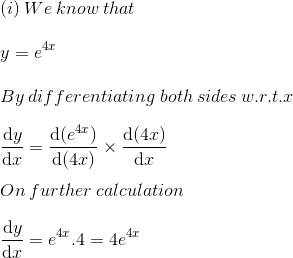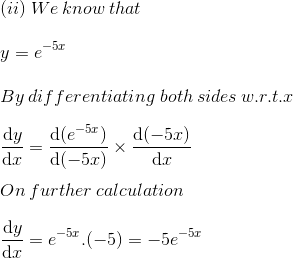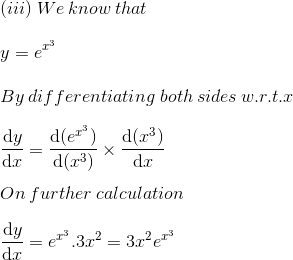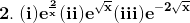Solution: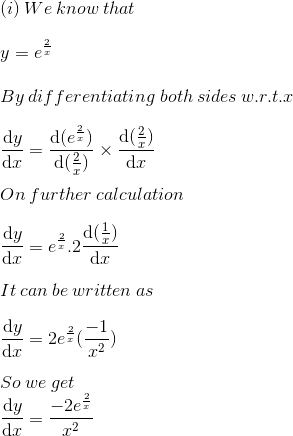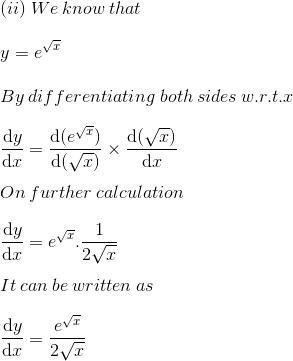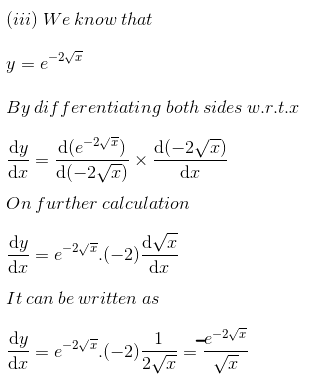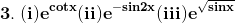Solution: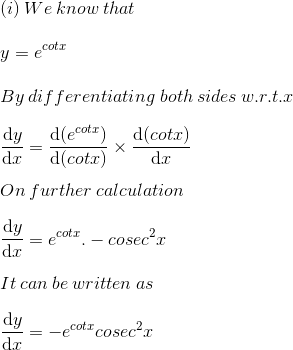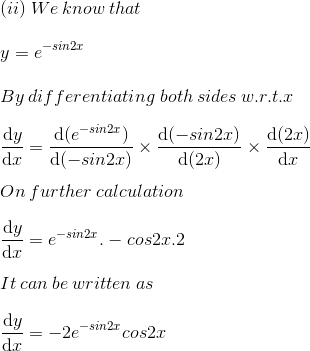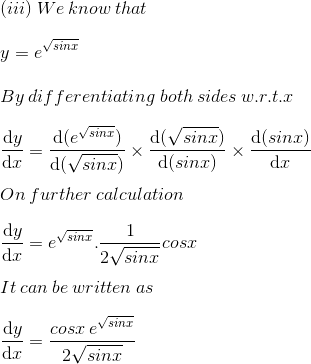4. (i) tan (logx)

(ii) log sec x

(iii) log sin x/2

Solution: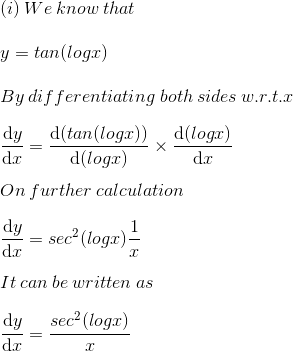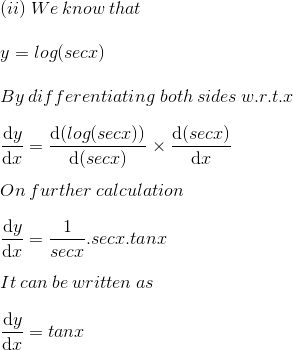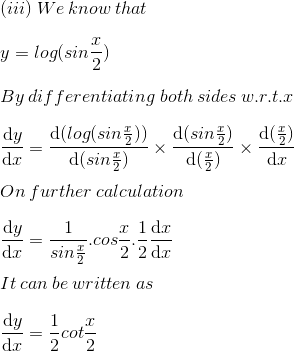5. (i) log3x

(ii) 2-x

(iii) 3x+2

Solution: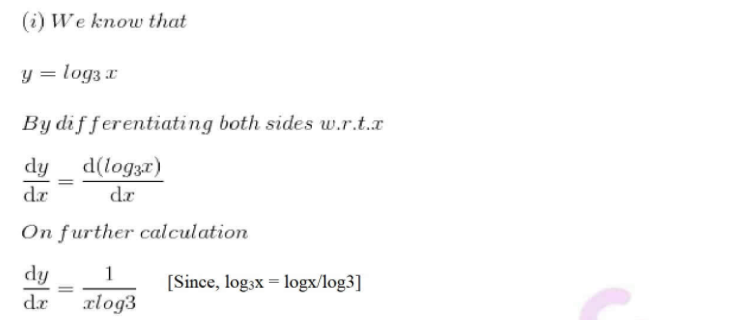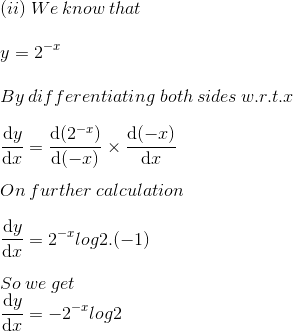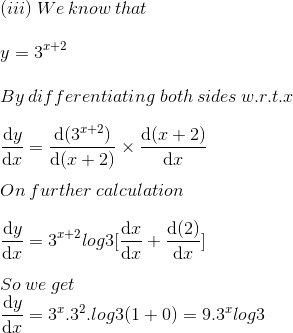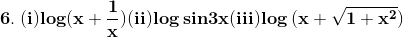Solution: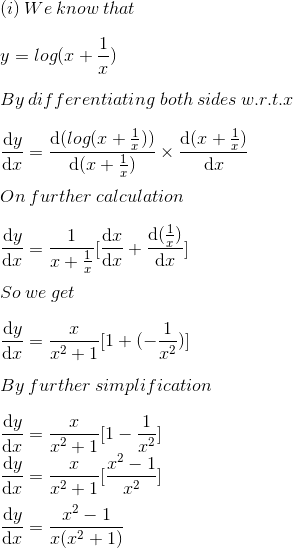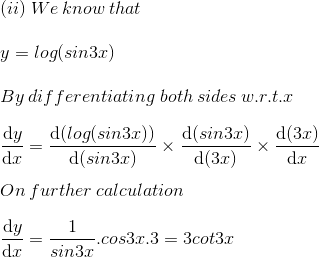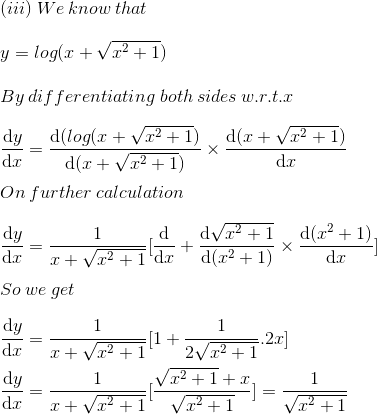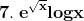Solution: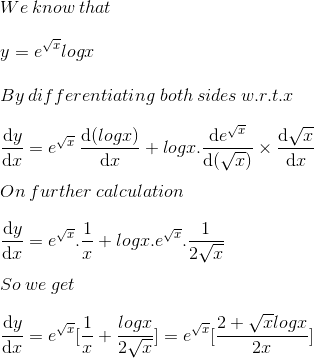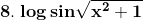Solution: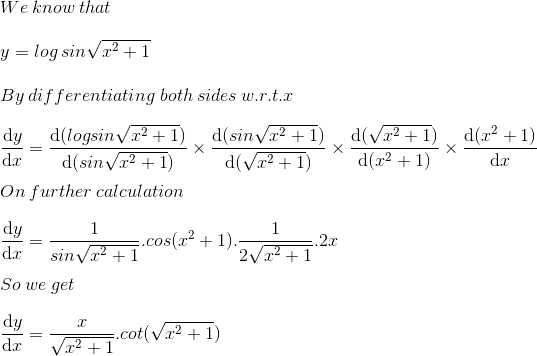9. e 2x sin3x

Solution: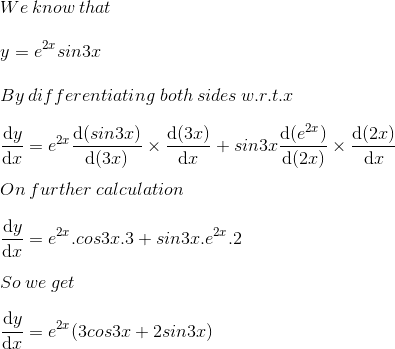10. e 3x cos2x

Solution: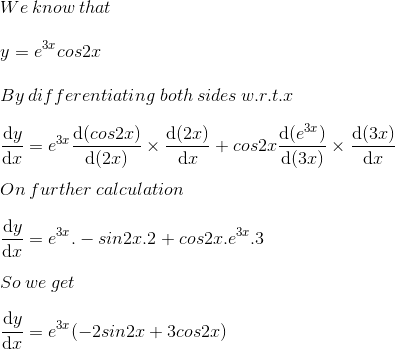11. e-5x cot4x

Solution: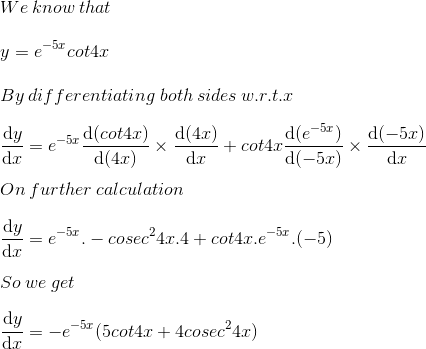#### 1 Comment

1. Vicky

This PDF. Is very useful for every students